# 用好组合索引，性能提升 10 倍不止！

0 62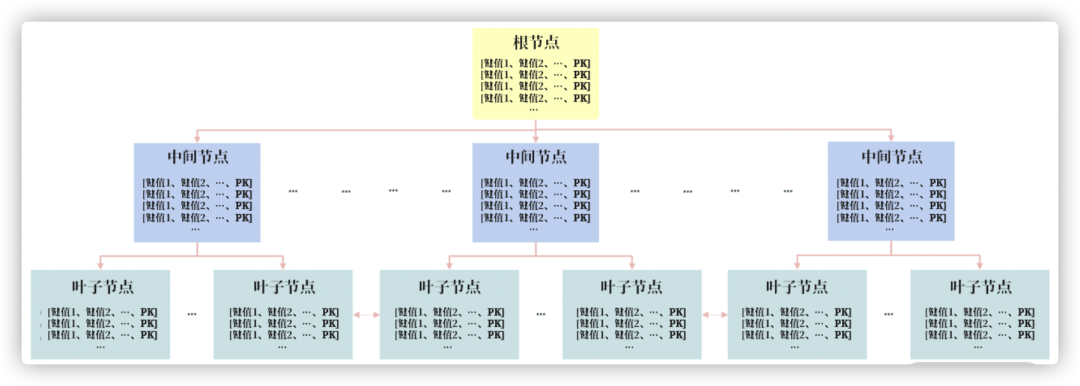``````create table test
(
id       int auto_increment primary key
name     varchar(50) null
workcode varchar(50) null
age      int         null
);

create index union_index on test (name workcode);
``````

``````select * from test  where  name = 'zhang' ;
select * from test  where  name = 'zhang' and workcode='20190169';
``````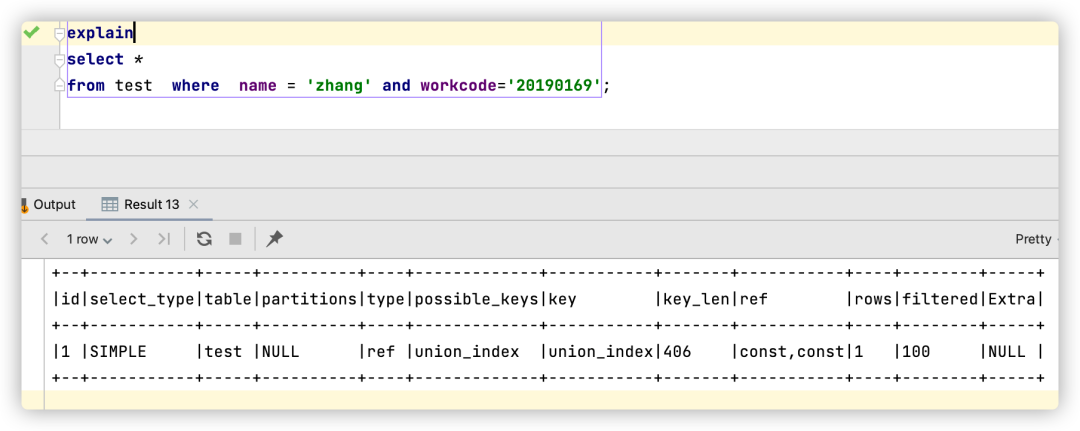``````select * from test where  workcode='20190169';
``````

``````select * from test  where  name = 'zhang' order by workcode;
``````

``````select * from test  where  workcode = '20190169' order by name ;
``````

``````CREATE TABLE t_opp_base
(
id                  int            primary key auto_increment
opp_code            varchar(50)    NOT NULL  -- 商机编码
opp_name            varchar(200)   NOT NULL
principal_user       varchar(50)    NOT NULL  -- 责任人
opp_status          char(1)        NOT NULL
opp_amount          decimal(15 2) NOT NULL
opp_date            date           NOT NULL
opp_priority        char(15)       NOT NULL
remark              varchar(79)    NOT NULL
KEY `idx_opp_code` (opp_code)
KEY `idx_principal_user` (principal_user)
);
``````

• 字段 id 是 INT 类型的主键；
• 字段 opp_code，principal_user 由于查询的场景比较多，所以添加了单字段索引
• 字段 opp_date、opp_status、opp_amount、opp_priority 用于商机的基本详情，分别表示商机时间、当前商机的状态、商机的总价值、商机的优先级。

``````select * from t_opp_base  where principal_user = 'javadaily' order by opp_date DESC
``````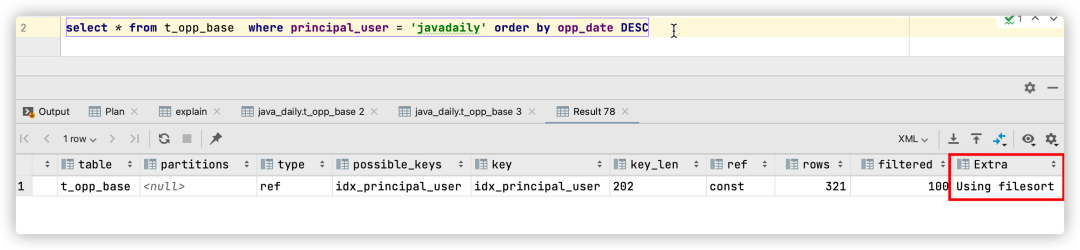``````create index idx_principal_oppdate
on t_opp_base (principal_useropp_date);
``````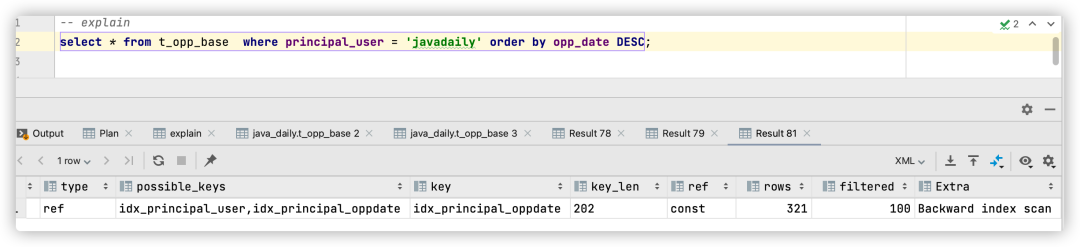“基础概念：SQL 需要二级索引查询得到主键值，然后再根据主键值搜索主键索引，最后定位到完整的数据。这一过程叫 回表。但是由于二级组合索引的叶子节点，包含索引键值和主键值，若查询的字段在二级索引的叶子节点中，则可直接返回结果，无需回表。这种通过组合索引避免回表的优化技术也称为 索引覆盖（Covering Index）。”

``````select principal_useropp_dateopp_amount from t_opp_base  where principal_user = 'javadaily' ;
``````

``````-> Index lookup on t_opp_base using idx_principal_oppdate (principal_user='javadaily')  (cost=312.51 rows=321) (actual time=0.452..0.908 rows=321 loops=1)
``````

“执行计划中显示执行成本 cost 为 312.51。（cost=312.51 表示的就是这条 SQL 当前的执行成本。不用关心 cost 的具体单位，你只需明白 cost 越小，开销越小，执行速度越快。）”

``````alter table t_opp_base add index
idx_principal_oppdate_amount(principal_useropp_dateopp_amount);
``````

``````-> Index lookup on t_opp_base using idx_principal_oppdate_amount (principal_user='javadaily')  (cost=41.52 rows=321) (actual time=0.149..0.337 rows=321 loops=1)
``````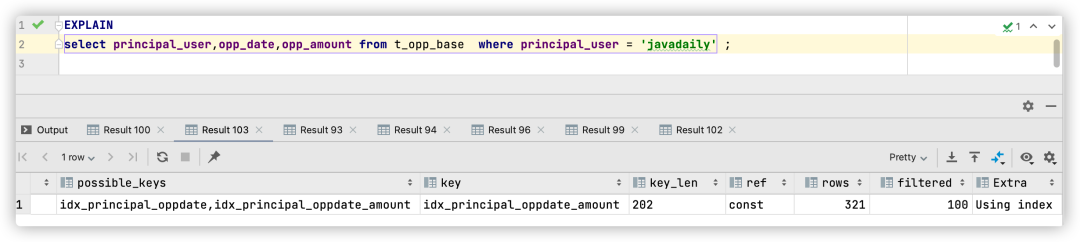``````select principal_usersum(opp_amount) from t_opp_base  group by principal_user;
``````

``````ALTER TABLE t_opp_base
drop INDEX idx_principal_oppdate_amount;
``````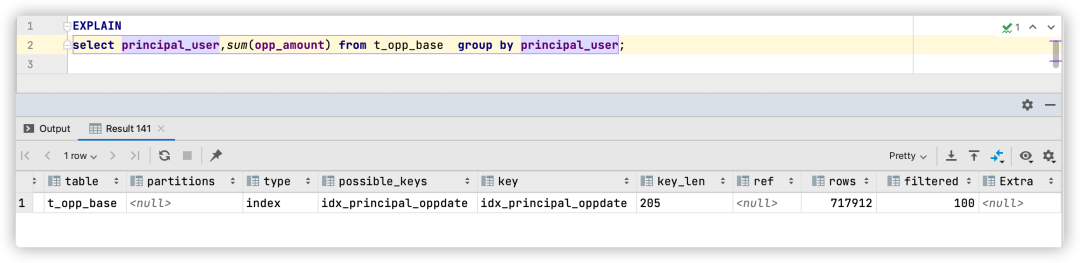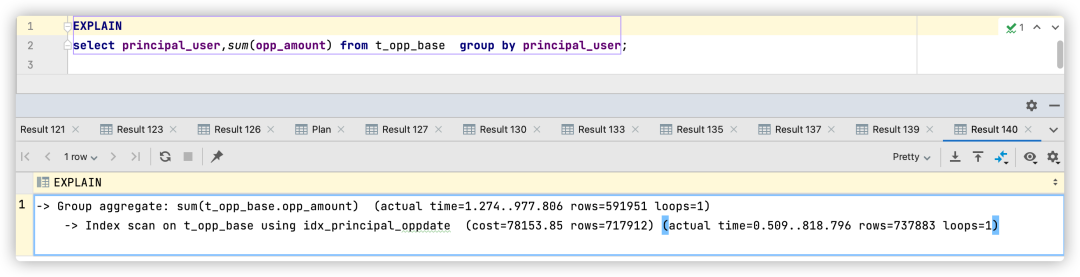``````alter table t_opp_base add index
idx_principal_oppdate_amount(principal_useropp_dateopp_amount);
``````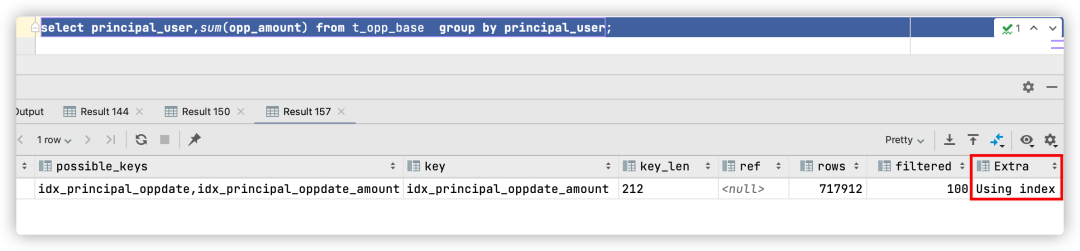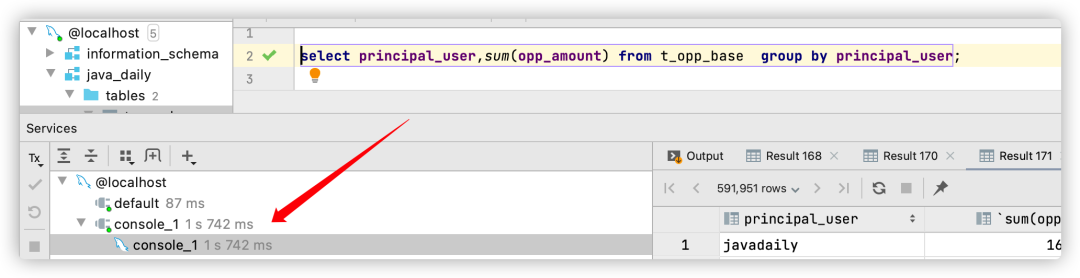• 覆盖多个查询条件，如（a，b）索引可以覆盖查询 a = ? 或者 a = ? and b = ?；
• 避免 SQL 的额外排序，提升 SQL 性能，如 WHERE a = ? ORDER BY b 这样的查询条件；
• 利用组合索引包含多个列的特性，可以实现索引覆盖技术，提升 SQL 的查询性能，用好索引覆盖技术，性能提升 10 倍不是难事。

Restful 如何进行版本控制 ,这 4 种方法你要掌握 59